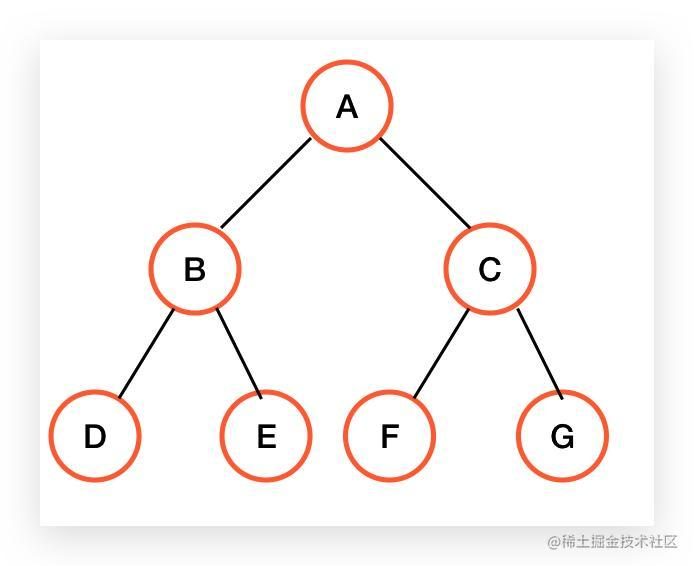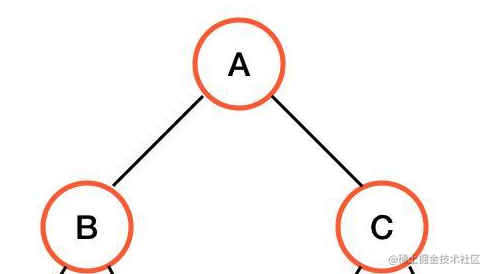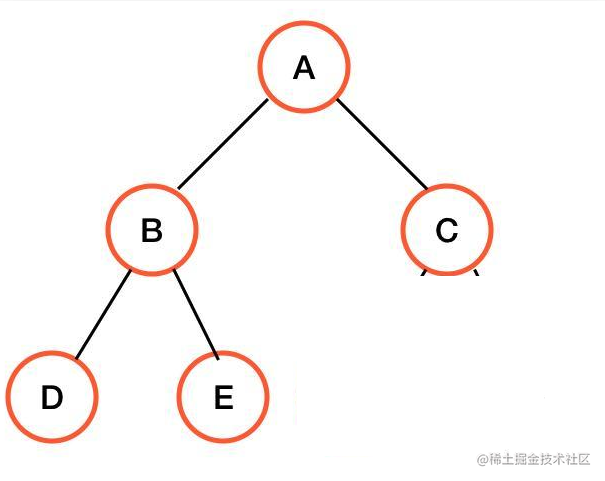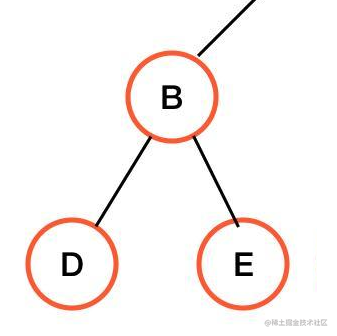[路飞]_一文彻底搞懂二叉树的前序、中序、后序遍历「这是我参与11月更文挑战的第9天，活动详情查看：2021最后一次更文挑战

例子概念递归B节点出现了子节点的时候， 三种遍历有什么不同呢？

前序遍历中序遍历B子树的中序结果是 D B E

后序遍历B子树的后序结果是 D E B

实现

前序遍历

/**
* Definition for a binary tree node.
* function TreeNode(val, left, right) {
*     this.val = (val===undefined ? 0 : val)
*     this.left = (left===undefined ? null : left)
*     this.right = (right===undefined ? null : right)
* }
*/
/**
* @param {TreeNode} root
* @return {number[]}
*/
function postorderTraversal(root) {
return root ? getNextNode(root) : [];
};

function getNextNode(node, result = []) {
result.push(node.val); // 前序遍历，把push当前节点放在前面
node.left && getNextNode(node.left, result);
node.right && getNextNode(node.right, result);
return result;
};

中序遍历

/**
* Definition for a binary tree node.
* function TreeNode(val, left, right) {
*     this.val = (val===undefined ? 0 : val)
*     this.left = (left===undefined ? null : left)
*     this.right = (right===undefined ? null : right)
* }
*/
/**
* @param {TreeNode} root
* @return {number[]}
*/
function postorderTraversal(root) {
return root ? getNextNode(root) : [];
};

function getNextNode(node, result = []) {
node.left && getNextNode(node.left, result);
result.push(node.val); // 中序遍历，把push当前节点放在中间
node.right && getNextNode(node.right, result);
return result;
};

后序遍历

/**
* Definition for a binary tree node.
* function TreeNode(val, left, right) {
*     this.val = (val===undefined ? 0 : val)
*     this.left = (left===undefined ? null : left)
*     this.right = (right===undefined ? null : right)
* }
*/
/**
* @param {TreeNode} root
* @return {number[]}
*/
function postorderTraversal(root) {
return root ? getNextNode(root) : [];
};

function getNextNode(node, result = []) {
node.left && getNextNode(node.left, result);
node.right && getNextNode(node.right, result);
result.push(node.val); // 后序遍历，把push当前节点放在最后面
return result;
};